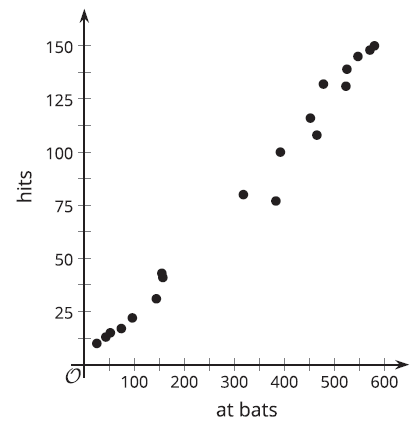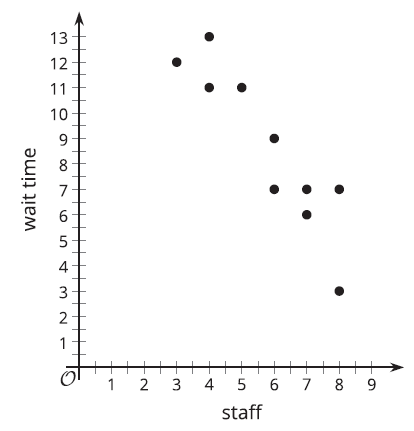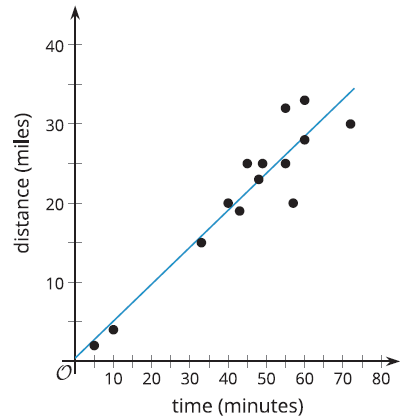# A.3.4 Practice Problems

The scatter plot shows the number of times a player came to bat and the number of hits they had.The scatter plot includes a point at (318, 80). Describe the meaning of this point in this situation.

The scatter plot shows the number of minutes people had to wait for service at a restaurant and the number of staff available at the time.A line that models the data is given by the equation y = -1.62x + 1.8, where y represents the wait time, and x represents the number of staff available. a. The slope of the line is -1.62. What does this mean in this situation? Is it realistic? b. The y-intercept is (0, 18). What does this mean in this situation? Is it realistic?

A taxi driver records the time required to complete various trips and the distance for each trip.The best fit line is given by the equation y = 0.467x + 0.417, where y represents the distance in miles, and x represents the time for the trip in minutes. a. Use the best fit line to estimate the distance for a trip that takes 20 minutes. Show your reasoning. b. Use the best fit line to estimate the time for a trip that is 6 miles long. Show your reasoning.